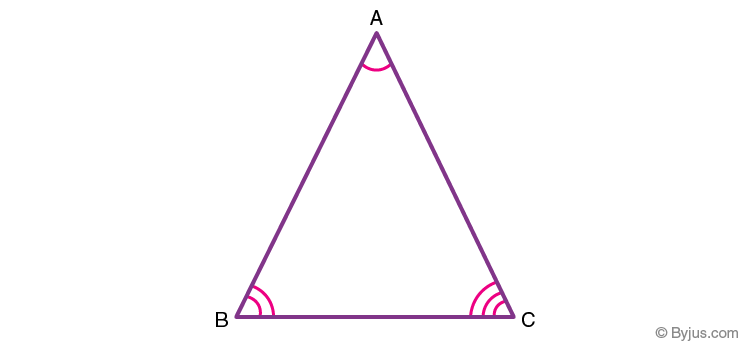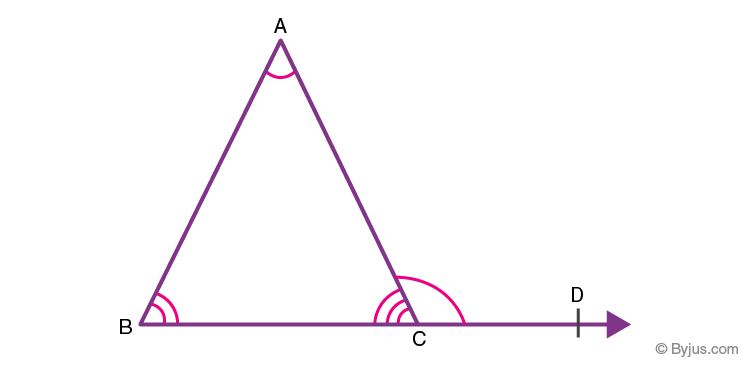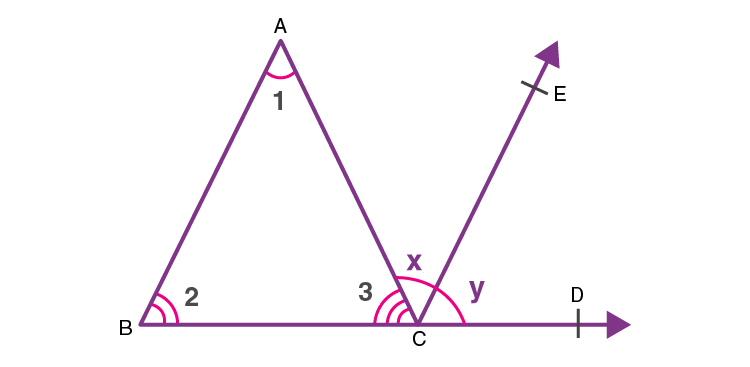# Triangles, Exterior Angle Theorem

The exterior angle theorem is one of the most fundamental theorems of triangles. Before we begin the discussion, let us have a look at what a triangle is. A polygon is defined as a plane figure bounded by a finite number of line segments to form a closed figure. Triangle is the polygon bounded by a least number of line segments, i.e. three. It has three edges and three vertices. Figure 1 below represents a triangle with three sides AB, BC, CA, and three vertices A, B and C. ∠ABC, ∠BCA and ∠CAB are the three interior angles of ∆ABC.Fig. 1 Triangle ABC

One of the basic theorems explaining the properties of a triangle is the exterior angle theorem. Let us discuss this theorem in detail.

## Exterior Angle Theorem

Statement: If a side of a triangle is produced, then the exterior angle so formed is equal to the sum of the two interior opposite angles.Fig. 2 Exterior Angle Theorem

The above statement can be explained using the figure provided as:

According to the Exterior Angle property of a triangle theorem, the sum of measures of ∠ABC and ∠CAB would be equal to the exterior angle ∠ACD.

General proof of this theorem is explained below:

Proof:

Consider a ∆ABC as shown in fig. 2, such that the side BC of ∆ABC is extended. A line, parallel to the side AB is drawn as shown in the figure.Fig. 3 Exterior Angle Theorem

 S. No Statement Reason 1. ∠CAB = ∠ACE ⇒∠1=∠x Pair of alternate angles(BA || CE) and (AC) is the transversal) 2. ∠ABC = ∠ECD ⇒∠2 = ∠y Corresponding angles (BA) ||(CE) and (BD) is the transversal) 3. ⇒∠1+∠2 = ∠x+∠y From statements 1 and 2 4. ∠x+∠y = ∠ACD From fig. 3 5. ∠1+∠2 = ∠ACD From statements 3 and 4

Thus, from the above statements, it can be seen that the exterior ∠ACD of ∆ABC is equal to the sum of two opposite interior angles i.e. ∠CAB and ∠ABC of the ∆ABC.

Hence proved.

## Frequently Asked Questions – FAQs

Q1

### What is the exterior angle theorem formula?

The below formulas can be stated from the exterior angle theorem.
The measure of exterior angle = Sum of two opposite interior angles’ measure
Q2

### What is the exterior angle Inequality Theorem?

According to the exterior angle inequality theorem, the measure of an exterior angle of a triangle is greater than either of its interior opposite angles.
Q3

### What is the exterior angle property?

If a side of a triangle is produced, then the exterior angle so formed is equal to the sum of the two interior opposite angles.
Q4

### Do all polygon exterior angles add up to 360?

Yes, the sum of exterior angles in a polygon is always added up to 360 degrees.
Q5

### What is the sum of the measures of the exterior angles of any triangle?

The sum of the measures of the exterior angles of any triangle is 360 degrees.
Test your knowledge on Exterior Angle Theorem